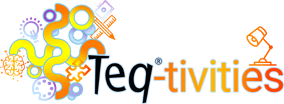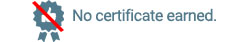## Math

### Instructional Quick Tips

###### No Certificates can be earned upon completing these videos.Reviewing Conic Sections

This video uses the "Conic Sections" Notebook file to help students review the four types of conice sections: circles, parabolas, ellipses and hyperbolas.  I demonstrate how a teacher can use a Notebook lesson file, Desmos Calculator website and animated gifs from Geogebra for visual modeling.

Non-Standard Units of Measure Kindergarten

During this activity students will measure various household objects using non-standard units of measure (paper clips).  This will be done both digitally with SMART Learning Suite Online and with manipulatives.

FOIL Method

Students will review the FOIL Method by working out problems in an online worksheet activity.  Then they will take an online assessment (SMART Response 2 Quiz through SLS Online).

In this activity, students will learn about Binary code and converting letters to binary. Students will use the knowledge to create a bracelet with 2 colored beads to represent their initials in Binary.

Find the Fraction

In this activity, students will be exploring and identifying equivalent fractions.Students will use Google Slides to review this concept and then they will test their knowledge by looking at fraction models and determine if they are equivalent or not equivalent. Students can collaborate with their peers by engaging in real-time discussion on Google Slides!

Calculating Mean, Median, and Mode

Students will find measures of central tendency, specifically mean, median, and mode. Students will learn how to calculate these meaures through the use of a random number generator, google forms, an interactive Peardeck presentation and a self correcting google sheet.

Triangle Offense with Sphero

Students will review a clip of the Chicago Bulls executing the Triangle Offense. They will pause the footage, determine what type of triangles the team is forming in that play, and program the Sphero robot (using either Scratch or Java script) to execute the triangles used in the play.

Evaluating Expressions

Students will evaluate expressions, both numerical and algebraic. Students will complete a Quizizz activity on this topic, either as a class or independently at the discretion of the teacher. As a closure students will create their own google slide with an expression and share with a partner for them to complete.

Congruence and Similarity

Students will determine if two or more shapes are congruent and similar. Students will flip, rotate, resize and move shapes on the canvas to determine congruence or similiarity.  Students will then describe the steps necessary to determine congruence or similiarity in the text box on the canvas.

Geometry Choice Board

Students will pick several topics from the choice board to complete using their knowledge of geometry, but also using other skills such as creative writing and illustrations.

Geometry Choice Board-SLSO

Students will pick several topics from the choice board to complete using their knowledge of geometry, but also using other skills such as creative writing and illustrations.

When teaching the Quadratic Formula to solve for x and graph parabolas (quadratic equations, we can present our Notebook file from SLS Online and give students inking tools to work out the process and graph the functions. Take a look!

Students will use the Sphero Mini to explore acute, right, and obtuse angles. Students will learn about and be able to identify the different types of angles. They will then measure the angles, program Sphero to drive over angles on a pre-made template and determine what type of angle it is. In this lesson, students will review different types of angles and have some coding fun with their Sphero Mini!

Bowling Alley Math

In this activity, students will engage in a Sphero Mini bowling activity with a math twist! Students will set up the bowling pins and use the Sphero to knock the pins down. Students will determine how many pins are left and they will subtract that number from the original number of pins. Additional "pins" or objects can be added on to make this activity more advanced. In addition, students can apply different math strategies such as addition, multiplication, and division to take this activity to the next level!

Probability with Ozobots

Students will learn about probability using Ozobots and their device free Color Coding.

Multiplication with Ozobots

Students will learn how to multiply numbers with the help of Ozobot color codes.

What's That Number?

In this activity, students will control Sphero to answer math questions. Teachers will set up number cards that will be placed throughout the classroom. Students will then be asked a question and students will drive the Sphero to the correct number card.

Sphero RVR - Right Triangle Paths

After studying interior and exterior angles of polygons, students will program their RVR to trace around the path of a large pentagon. This will involve speed and heading settings in the Sphero EDU blocks.

Sphero RVR - Pentagon Paths

After studying interior and exterior angles of polygons, students will program their RVR to trace around the path of a large pentagon. This will involve speed and heading settings in the Sphero EDU blocks.

Sphero RVR - Angle of Incline

Students studying trigonometry will apply their knowledge of solving a triangle to answer this question: What is the steepest angle of incline that the RVR can travel upwards? Students will work in pairs using a ramp and measuring tape to draw a diagram and calculate the angle, test driving the RVR in several trials.

Sphero RVR - Trig Triangle Paths

The teacher will print and hand out several triangle pictures to each group of students. They will calculate the side lengths and angle measures with only limited given information. This lesson involves using trig functions and formulas studied in Algebra 1 or 2 and is a great application of "Solving a Triangle". Once the triangle is solved, students will code the robot to move along the triangle's path.

Rolling Down the Number Line Fraction Edition for Sphero SPRK and Bolt

In this activity students will use the Sphero SPRk+ or Bolt to practice fractions.  They will do this by rolling their robot the the assigned fraction onthe number line.

### Certificate Bearing Courses

#### Digital Online Certifications

Below are some popular Digital Teacher Certifications that are available as a series of online courses. Click on a logo to start your certification track today!